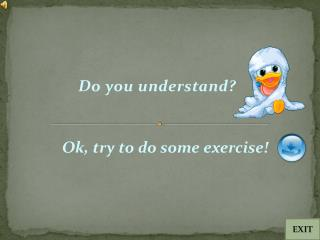DownloadDownload PresentationDo you understand?

# Do you understand?

Télécharger la présentation## Do you understand?

- - - - - - - - - - - - - - - - - - - - - - - - - - - E N D - - - - - - - - - - - - - - - - - - - - - - - - - - -
##### Presentation Transcript

1. Do you understand? Ok, try to do some exercise! EXIT

2. 1. A CCD camera chip of dimensions 7×7mm, and having 1024×1024 elements, is focused on a square, flat area, located 0.5 m away. How many line pairs per mm will this camera be able to resolve? The camera is equipped with a 35-mm lens. (Hint: Model the imaging process as in Fig.2.3, with the focal length of the camera lens substituting for the focal length of the eye.)

3. 2. Propose a set of gray-level-slicing transformations capable of producing all the individual bit planes of an 8-bit monochrome image.(For example, a transformation function with the property T(r)=0 for r in the range [0,127], and T(r)=255 for r in the range [128,255] produces an image of the 7th bit plane in an 8-bit image.)

4. 3. In a given application an averaging mask is applied to input image to reduce noise, and then a Laplacian mask is applied to enhance small details. Would the result be the same if the order of these operations were reversed?

5. 4. Show that if a filter transfer function H(u, v) is real and symmetric, then the corresponding spatial domain filter h(x, y) also is real and symmetric. • 5. Develop an algorithm for converting a one-pixel-thick m-path to a 4-path.

6. 6. During acquisition, an image undergoes uniform linear motion in the vertical direction for a time T1, The direction of motion then switches to the horizontal direction for a time interval T2. Assuming that the time it takes the image to change direction is negligible, and that shutter opening and closing times are negligible also, give an expression for the blurring function, H(u, v).

7. 7. Propose a technique for detecting gaps of length ranging between 1 and L pixels in line segments of a binary image. Assume that the lines are 1 pixel thick. Base your technique on 8-neighbor connectivity analysis, rather than attempting to construct masks for detecting the gaps.

8. 8. Prove the validity of the convolution theorem. For simplicity, limit your development to continuo’s functions of one variable. • 9. How many different shades of gray are there in a color RGB system in which each RGB image is an 8-bit image?